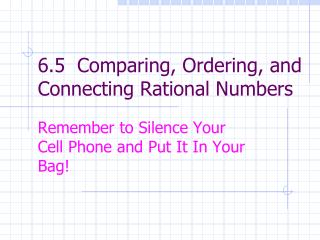DownloadDownload Presentation6.5 Comparing, Ordering, and Connecting Rational Numbers

# 6.5 Comparing, Ordering, and Connecting Rational Numbers

Télécharger la présentation## 6.5 Comparing, Ordering, and Connecting Rational Numbers

- - - - - - - - - - - - - - - - - - - - - - - - - - - E N D - - - - - - - - - - - - - - - - - - - - - - - - - - -
##### Presentation Transcript

1. 6.5 Comparing, Ordering, and Connecting Rational Numbers Remember to Silence Your Cell Phone and Put It In Your Bag!

2. Comparing Rational Numbers (in fraction form) • Models • For , where b>0, iff a<c. • For , where b>0 and d>0, iff ad<bc.

3. Definition of Less Than for Rational Numbers • For , iff a rational number exists such that . • If , then .

4. Be able to: • Order rational numbers in fraction form • Order rational numbers in decimal form

5. Denseness Property for Rational Numbers • Between any two rational numbers there exists at least one rational number. • Be able to find rational numbers between two given rational numbers • Fraction Form • Decimal Form

6. Converting Decimals to Fractions Be able to: • Terminating Decimals  Fractions • Repeating Decimals  Fractions

7. You may omit Scientific Notation

8. Relationships Among these Sets of Numbers N  W  I  Q Q I W N

9. What numbers are not Rational Numbers? • Every rational number can be expressed as a terminating or repeating decimal. • Numbers which cannot be expressed as either repeating or terminating decimals are not rational numbers.

10. The Set of Irrational Numbers • Real numbers which cannot be expressed as either repeating or terminating decimals. • Examples:

11. The Set of Real Numbers {Rational Numbers} ⋃{Irrational Numbers} = {Real Numbers} • Note – The set of rational numbers and the set of irrational numbers are disjoint sets.

12. What numbers are not Real numbers? • _____________ numbers are not real numbers. • Examples: